## Math Puzzles is a free logic math game & an efficient brain teaser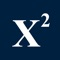# Math Puzzles & Riddles Game

by Oksana Vengrovska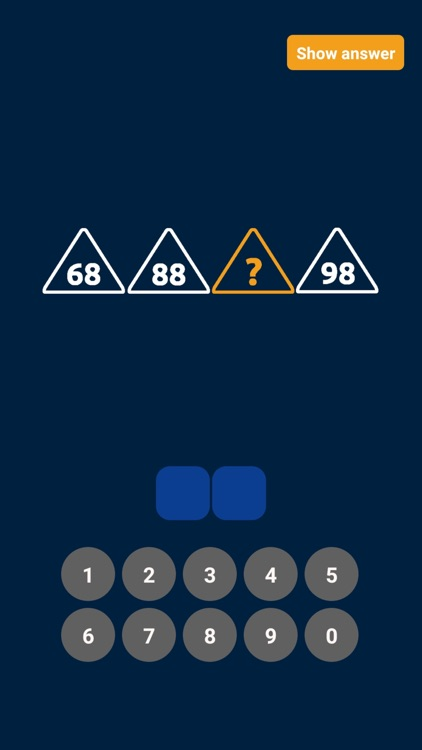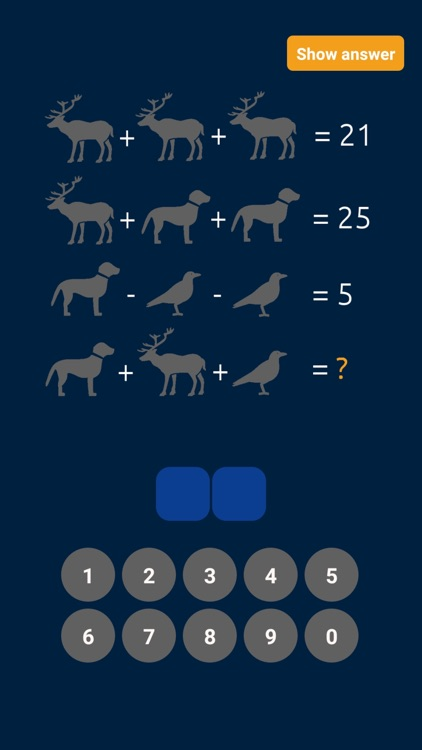Math Puzzles is a free logic math game & an efficient brain teaser. With Math Puzzles, it is fun & easy to practice math & solve math problems. Math Puzzles is a free math quiz with no extra costs hidden.### App Details

Version
1.0.7
Rating
(1)
Size
81Mb
Genre
Education Puzzle Trivia
Last updated
April 4, 2021
Release date
March 14, 2021

### App Screenshots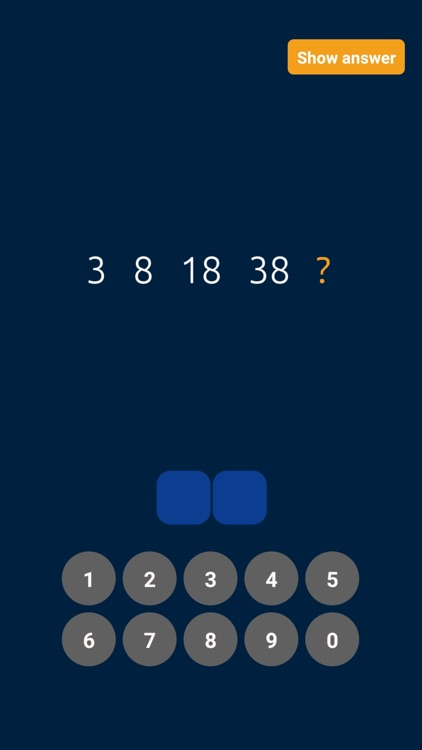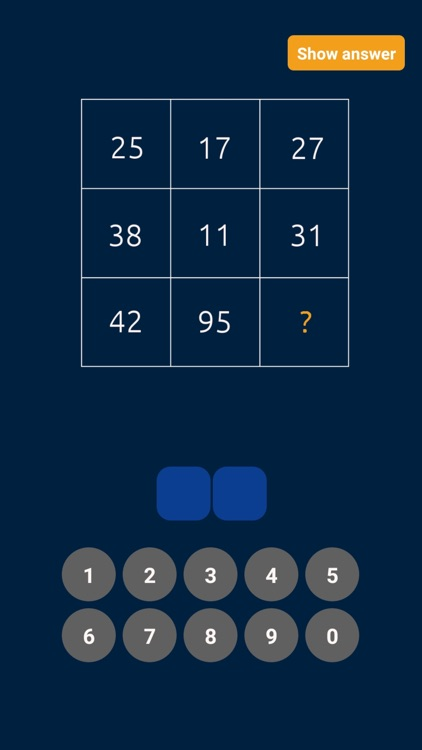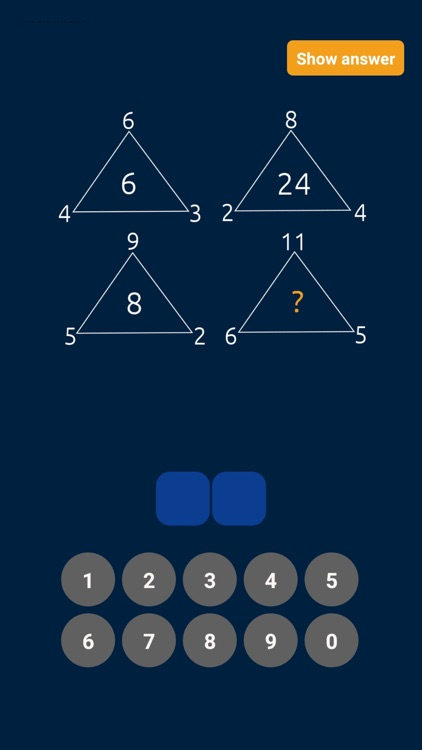### App Store Description

Math Puzzles is a free logic math game & an efficient brain teaser. With Math Puzzles, it is fun & easy to practice math & solve math problems. Math Puzzles is a free math quiz with no extra costs hidden.

Math Puzzles & Riddles features:
- Interesting math tasks. Solve math problems & enjoy an exciting math workout.
- Engaging logic puzzles. Math Puzzles is a free brain teaser for all who love brain games, solving logic puzzles, practicing math & solving math problems.
- Unique math riddles. Math Puzzles is one of the few problem solving games offering unique math riddles. You will not find similar math tasks anywhere else.